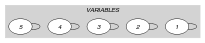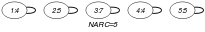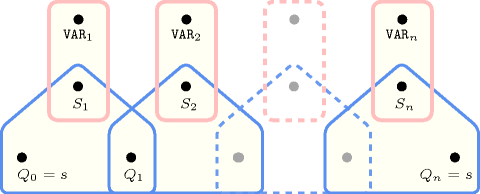## 5.31. arith

Origin

Used in the definition of several automata

Constraint

$\mathrm{𝚊𝚛𝚒𝚝𝚑}\left(\mathrm{𝚅𝙰𝚁𝙸𝙰𝙱𝙻𝙴𝚂},\mathrm{𝚁𝙴𝙻𝙾𝙿},\mathrm{𝚅𝙰𝙻𝚄𝙴}\right)$

Synonym

$\mathrm{𝚛𝚎𝚕}$.

Arguments
 $\mathrm{𝚅𝙰𝚁𝙸𝙰𝙱𝙻𝙴𝚂}$ $\mathrm{𝚌𝚘𝚕𝚕𝚎𝚌𝚝𝚒𝚘𝚗}\left(\mathrm{𝚟𝚊𝚛}-\mathrm{𝚍𝚟𝚊𝚛}\right)$ $\mathrm{𝚁𝙴𝙻𝙾𝙿}$ $\mathrm{𝚊𝚝𝚘𝚖}$ $\mathrm{𝚅𝙰𝙻𝚄𝙴}$ $\mathrm{𝚒𝚗𝚝}$
Restrictions
 $\mathrm{𝚛𝚎𝚚𝚞𝚒𝚛𝚎𝚍}$$\left(\mathrm{𝚅𝙰𝚁𝙸𝙰𝙱𝙻𝙴𝚂},\mathrm{𝚟𝚊𝚛}\right)$ $\mathrm{𝚁𝙴𝙻𝙾𝙿}\in \left[=,\ne ,<,\ge ,>,\le \right]$
Purpose

Enforce for all variables $\mathrm{𝚟𝚊𝚛}$ of the $\mathrm{𝚅𝙰𝚁𝙸𝙰𝙱𝙻𝙴𝚂}$ collection to have $\mathrm{𝚟𝚊𝚛}\mathrm{𝚁𝙴𝙻𝙾𝙿}\mathrm{𝚅𝙰𝙻𝚄𝙴}$.

Example
$\left(〈4,5,7,4,5〉,<,9\right)$

The $\mathrm{𝚊𝚛𝚒𝚝𝚑}$ constraint holds since all values of the collection $〈4,5,7,4,5〉$ are strictly less than 9.

Typical
 $|\mathrm{𝚅𝙰𝚁𝙸𝙰𝙱𝙻𝙴𝚂}|>1$ $\mathrm{𝚁𝙴𝙻𝙾𝙿}\in \left[=\right]$
Symmetries
• Items of $\mathrm{𝚅𝙰𝚁𝙸𝙰𝙱𝙻𝙴𝚂}$ are permutable.

• An occurrence of a value of $\mathrm{𝚅𝙰𝚁𝙸𝙰𝙱𝙻𝙴𝚂}.\mathrm{𝚟𝚊𝚛}$ can be replaced by any value of $\mathrm{𝚅𝙰𝚁𝙸𝙰𝙱𝙻𝙴𝚂}.\mathrm{𝚟𝚊𝚛}$.

Arg. properties

Contractible wrt. $\mathrm{𝚅𝙰𝚁𝙸𝙰𝙱𝙻𝙴𝚂}$.

Systems

eq in Choco, neq in Choco, geq in Choco, gt in Choco, leq in Choco, lt in Choco, rel in Gecode, #< in SICStus, #=< in SICStus, #> in SICStus, #>= in SICStus, #= in SICStus, #\= in SICStus.

Used in

generalisation: $\mathrm{𝚊𝚛𝚒𝚝𝚑}_\mathrm{𝚘𝚛}$ ($\mathrm{𝚟𝚊𝚛𝚒𝚊𝚋𝚕𝚎}$ $\mathrm{𝚁𝙴𝙻𝙾𝙿}$ $\mathrm{𝚅𝙰𝙻𝚄𝙴}$ replaced by $\mathrm{𝚟𝚊𝚛𝚒𝚊𝚋𝚕𝚎}$ $\mathrm{𝚁𝙴𝙻𝙾𝙿}$ $\mathrm{𝚅𝙰𝙻𝚄𝙴}$ $\vee$ $\mathrm{𝚟𝚊𝚛𝚒𝚊𝚋𝚕𝚎}$ $\mathrm{𝚁𝙴𝙻𝙾𝙿}$ $\mathrm{𝚅𝙰𝙻𝚄𝙴}$).

Keywords
Cond. implications

$\mathrm{𝚊𝚛𝚒𝚝𝚑}\left(\mathrm{𝚅𝙰𝚁𝙸𝙰𝙱𝙻𝙴𝚂},\mathrm{𝚁𝙴𝙻𝙾𝙿},\mathrm{𝚅𝙰𝙻𝚄𝙴}\right)$

with  $\mathrm{𝚁𝙴𝙻𝙾𝙿}\in \left[<\right]$

and   $\mathrm{𝚖𝚒𝚗𝚟𝚊𝚕}$$\left(\mathrm{𝚅𝙰𝚁𝙸𝙰𝙱𝙻𝙴𝚂}.\mathrm{𝚟𝚊𝚛}\right)\ge 0$

implies $\mathrm{𝚛𝚊𝚗𝚐𝚎}_\mathrm{𝚌𝚝𝚛}$$\left(\mathrm{𝚅𝙰𝚁𝙸𝙰𝙱𝙻𝙴𝚂},\mathrm{𝙲𝚃𝚁},𝚁\right)$

when  $\mathrm{𝙲𝚃𝚁}\in \left[<\right]$.

Arc input(s)

$\mathrm{𝚅𝙰𝚁𝙸𝙰𝙱𝙻𝙴𝚂}$

Arc generator
$\mathrm{𝑆𝐸𝐿𝐹}$$↦\mathrm{𝚌𝚘𝚕𝚕𝚎𝚌𝚝𝚒𝚘𝚗}\left(\mathrm{𝚟𝚊𝚛𝚒𝚊𝚋𝚕𝚎𝚜}\right)$

Arc arity
Arc constraint(s)
$\mathrm{𝚟𝚊𝚛𝚒𝚊𝚋𝚕𝚎𝚜}.\mathrm{𝚟𝚊𝚛}\mathrm{𝚁𝙴𝙻𝙾𝙿}\mathrm{𝚅𝙰𝙻𝚄𝙴}$
Graph property(ies)
$\mathrm{𝐍𝐀𝐑𝐂}$$=|\mathrm{𝚅𝙰𝚁𝙸𝙰𝙱𝙻𝙴𝚂}|$

Graph model

Parts (A) and (B) of Figure 5.31.1 respectively show the initial and final graph associated with the Example slot. Since we use the $\mathrm{𝐍𝐀𝐑𝐂}$ graph property, the loops of the final graph are stressed in bold.

##### Figure 5.31.1. Initial and final graph of the $\mathrm{𝚊𝚛𝚒𝚝𝚑}$ constraint(a) (b)
Automaton

Figure 5.31.2 depicts the automaton associated with the $\mathrm{𝚊𝚛𝚒𝚝𝚑}$ constraint. To each variable ${\mathrm{𝚅𝙰𝚁}}_{i}$ of the collection $\mathrm{𝚅𝙰𝚁𝙸𝙰𝙱𝙻𝙴𝚂}$ corresponds a 0-1 signature variable ${S}_{i}$. The following signature constraint links ${\mathrm{𝚅𝙰𝚁}}_{i}$ and ${S}_{i}$: ${\mathrm{𝚅𝙰𝚁}}_{i}\mathrm{𝚁𝙴𝙻𝙾𝙿}\mathrm{𝚅𝙰𝙻𝚄𝙴}⇔{S}_{i}$. The automaton enforces for each variable ${\mathrm{𝚅𝙰𝚁}}_{i}$ the condition ${\mathrm{𝚅𝙰𝚁}}_{i}\mathrm{𝚁𝙴𝙻𝙾𝙿}\mathrm{𝚅𝙰𝙻𝚄𝙴}$.

##### Figure 5.31.2. Automaton of the $\mathrm{𝚊𝚛𝚒𝚝𝚑}$ constraint##### Figure 5.31.3. Hypergraph of the reformulation corresponding to the automaton of the $\mathrm{𝚊𝚛𝚒𝚝𝚑}$ constraint